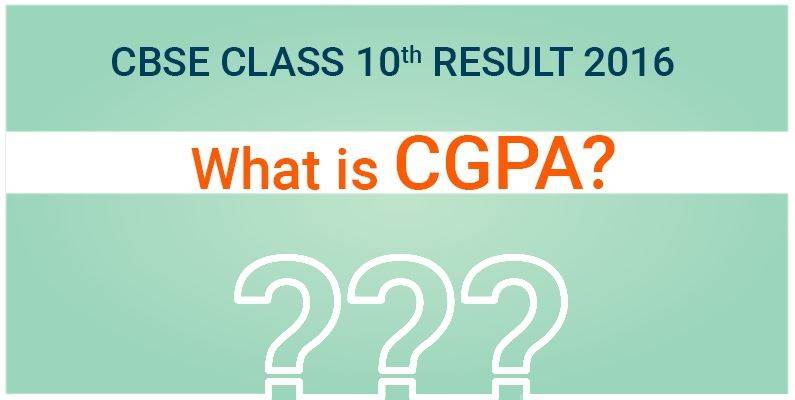# What is CGPA? Know here!Class 10th CBSE board results are expected to be announced on May 21st. Students can download their result from CBSE’s official site cbse.nic.in.

Lakh of student appears for CBSE examination. Since the year 2011, CBSE has started the system of announcing the results in CGPA. Many students and parents are confused as how to calculate the accurate percentage of the student.

What is CGPA? CGPA means the average obtained Grade Points in the all the subject – additional 6th subject is excluded.  CGPA shouldn’t be confused with the percentage. There is different method to calculate the percentage. You can multiply the overall CGPA to calculate the percentage with 9.5. Why is it multiplied with 9.5 only? CBSE board calculated the marks of all the 91 – 100 scorer and took an average of the score. That average was 95 percent.

So an indicative equivalent percentage of the grade point can be calculated as:

Subject wise percentage =  Grade point (GP) obtained by the student x 9.5
And the overall percentage of marks = 9.5 x CGPA

The board have an disclaimer that the percentage is only approximation and not exact percentage.
Let’s take an example to convert CGPA to GPA:
Suppose the student scored
Subject 1 – 9
Subject 2 – 10
Subject 3 – 9
Subject 4 – 7.5
Subject 5- 8

So now the sum of the GPs will be : 9+8+7.5+9+10= 43.5
Dividing the sum of all the GPs with 5 will be 43.5 / 5= 8.7

8.7 is the overall indicative percentage of marks
Now, to find out the  approximate percentage of marks, just multiply the CGPA with 9.5 = 9.7 x 9.5 = 82.65

So now that you know the trick, calculate your percentage and comment below!

Team Byju’s wishes you All the Best.

## CBSE Results 2016

#### Register Here To Get Your Results

* Subject to availability of result from respective Boards.